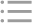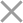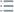## Fill out the form in 2 simple steps below:

Step 1

Step 2
** By clicking this button you agree to our Privacy Policy statementRequest our prices
--Select currency--
USD
EUR
* By clicking this button you agree to our Privacy Policy statementFree PVS‑Studio license for Microsoft MVP specialists
* By clicking this button you agree to our Privacy Policy statementTo get the licence for your open-source project, please fill out this form
* By clicking this button you agree to our Privacy Policy statementI am interested to try it on the platforms:
 Windows Linux macOS PVS-Studio for .NET Core JetBrains Rider
* By clicking this button you agree to our Privacy Policy statementMessage submitted.

Your message has been sent. We will email you at

check your Spam/Junk folder and click the "Not Spam" button for our message.
This way, you won't miss messages from our team in the future.

>
>
>
V3134. Shift by N bits is greater than …Introduction
Analyzing projects
On Windows
On Linux and macOS
Cross-platform
IDE
Build systems
Game Engines
Continuous use of the analyzer in software development
Deploying the analyzer in cloud Continuous Integration services
Managing analysis results
Analyzer diagnostics
General Analysis (C++)
General Analysis (C#)
General Analysis (Java)
Micro-Optimizations (C++)
Micro-Optimizations (C#)
Diagnosis of 64-bit errors (Viva64, C++)
Customer specific requests (C++)
MISRA errors
AUTOSAR errors
OWASP errors (C++)
OWASP errors (C#)
OWASP errors (Java)
Problems related to code analyzerContents

# V3134. Shift by N bits is greater than the size of type.

Feb 10 2017

The analyzer detected a likely error that has to do with shifting a value of an integer number by 'N' bits, 'N' being greater than the length of this type in bits.

Consider the following example:

``````UInt32 x = ....;
UInt32 y = ....;
UInt64 result = (x << 32) + y;``````

The programmer intended to form a 64-bit value from two 32-bit ones by shifting 'x' by 32 bits and adding the most significant and the least significant parts. However, as 'x' is a 32-bit value at the moment when the shift operation is performed, shifting by 32 bits will be equivalent to shifting by 0 bits, which will lead to an incorrect result.

This is what the fixed version of the code could look like:

``````UInt32 x = ....;
UInt32 y = ....;
UInt64 result = ((UInt64)x << 32) + y;``````

Now consider the following example from a real project:

``````static long GetLong(byte[] bits)
{
return ((bits & 0xff) << 0)
| ((bits & 0xff) << 8)
| ((bits & 0xff) << 16)
| ((bits & 0xff) << 24)
| ((bits & 0xff) << 32)
| ((bits & 0xff) << 40)
| ((bits & 0xff) << 48)
| ((bits & 0xff) << 56);
}``````

In the 'GetLong' method, an array of bytes is cast to a 64-bit value. Since bitwise shift operations are defined only for 32-bit and 64-bit values, each byte will be implicitly cast to 'Int32'. The bitwise shift range for a 32-bit value is [0..31], so the cast will be performed correctly only for the first 4 bytes of the array.

If the byte array was formed from a 64-bit value (for example 'Int64.MaxValue'), then casting the array back to Int64 using this method will result in an error if the original value was beyond the range [Int32.MinValue....Int32.MaxValue].

For a better understanding of this, let's see what happens when this code is executed over the value '289077008695033855' as an example. When cast to an array of bytes, this value will look as follows:

``289077008695033855 => [255, 255, 255, 255, 1, 2, 3, 4]``

After passing this array to method 'GetLong', each byte will be implicitly cast to Int32 before executing the shift operation. Let's shift each element separately, so we can see where the problem is:As you can see, each shift is performed over a 32-bit value, which causes range overlapping and, therefore, leads to an incorrect result. This happens because when attempting to shift a 32-bit value by more than 32 bits, they are shifted in a circle (shifting by 32, 40, 48, and 56 bits is equivalent to shifting by 0, 8, 16, and 24 bits respectively).

The fixed version of the code above could look like this:

``````static long GetLong(byte[] bits)
{
return ((long)(bits & 0xff) << 0)
| ((long)(bits & 0xff) << 8)
| ((long)(bits & 0xff) << 16)
| ((long)(bits & 0xff) << 24)
| ((long)(bits & 0xff) << 32)
| ((long)(bits & 0xff) << 40)
| ((long)(bits & 0xff) << 48)
| ((long)(bits & 0xff) << 56);
}``````

If we now examine each shift operation separately, we will see that the shifts are performed over 64-bit values, which prevents range overlapping.This diagnostic is classified as:

 You can look at examples of errors detected by the V3134 diagnostic.